# #608 LED Current Control

Using an Arduino PWM signal for smooth current-controlled LED dimming with a current mirror.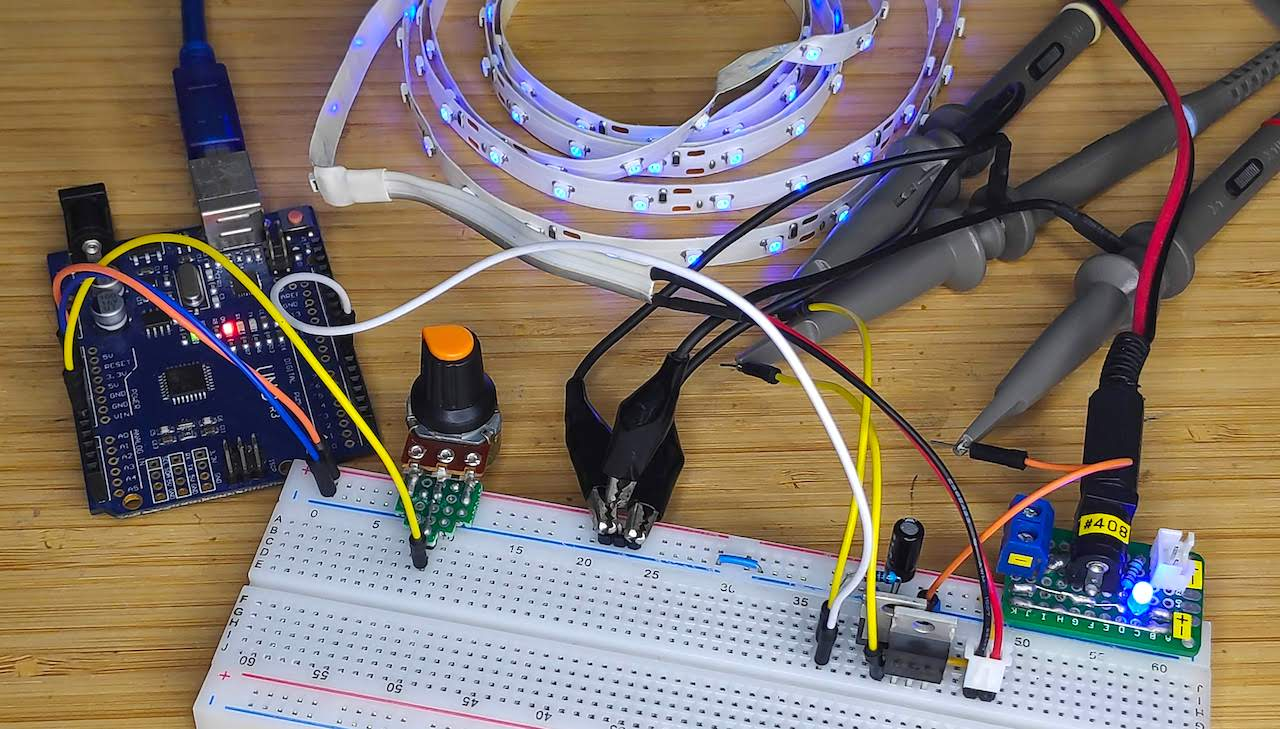PWM outputs are convenient for LED dimming under the control of a microprocessor. The main downside with direct PWM control is flicker. This is especially true when using an Arduino, which has a default PWM frequency of only 490 to 1000 Hz (depending on pin and model).

The best flicker-free LED control is achieved with current control. Fixed current circuits are straight-forward, as the necessary current limiting resistors/control circuitry can be pre-calculated.

However variable current control (i.e. dimming) is a little more challenging with a microcontroller, as they can’t directly provide current control.

This project tests a simple current control circuit that provides variable current (brightness) control governed by a microprocessor.

## Construction

The circuit comprises 3 significant control mechanisms:

• the pair of n-channel MOSFETs (IRF3205) implement a current-mirror:
• Q1 is maintained in saturation mode (since gate is shorted to drain)
• Q1 and Q2 always have the same gate-to-source voltage (as both gates and both sources are linked)
• assuming Q1 and Q2 are identical devices, their drain currents will be equal regardless of the voltage at the drain of Q2
• thus the current we apply to Q1 will control the current flowing through Q2 (thus the LEDs attached to Q2)
• the RC filter on the pin 6 PWM output smooths the output to essentially a fixed voltage level. The voltage level will determine the current to Q1
• the variable resistor provides an analog input for the Arudino - this is used to set the target brightness i.e. the PWM duty cycle on pin 6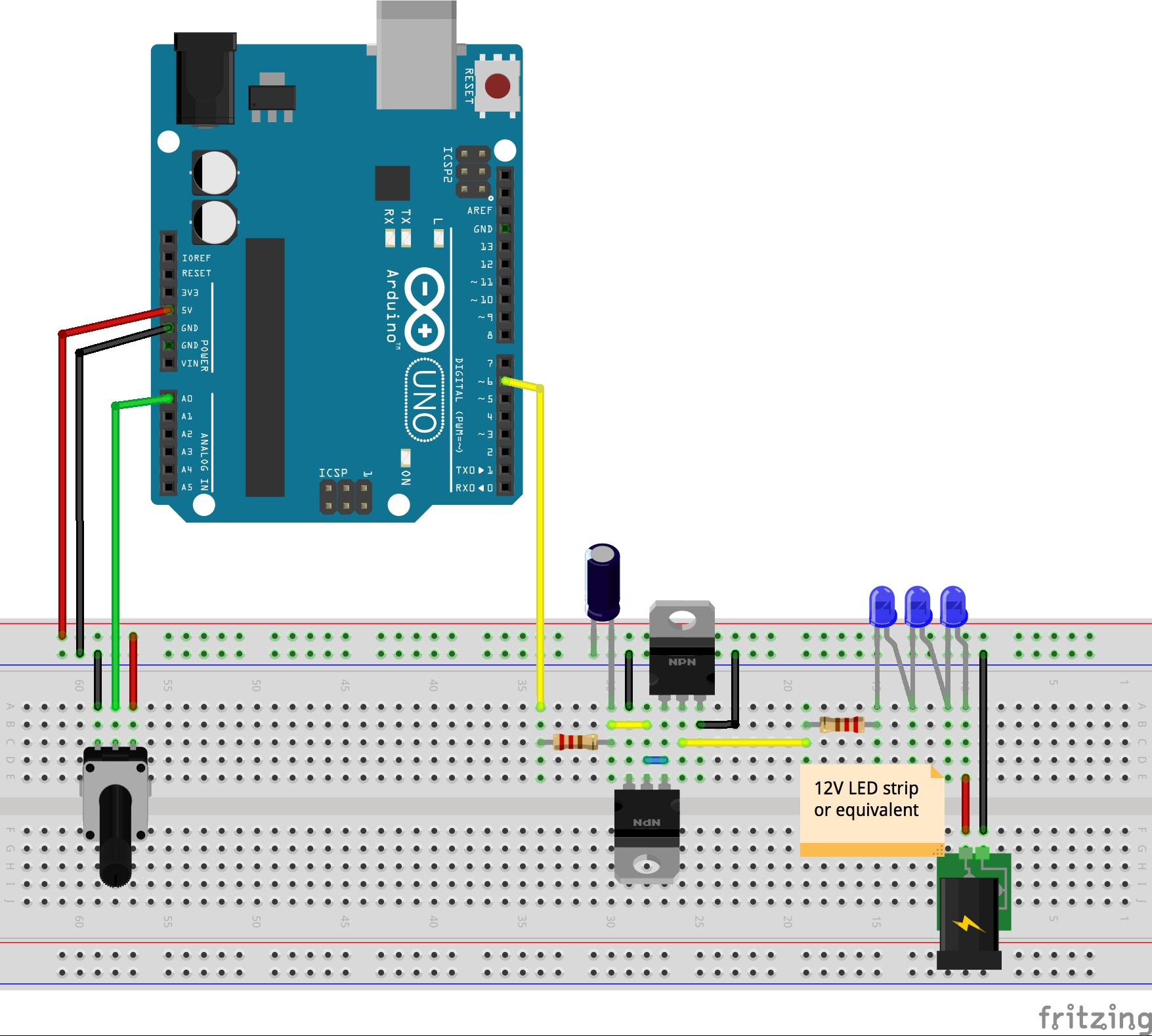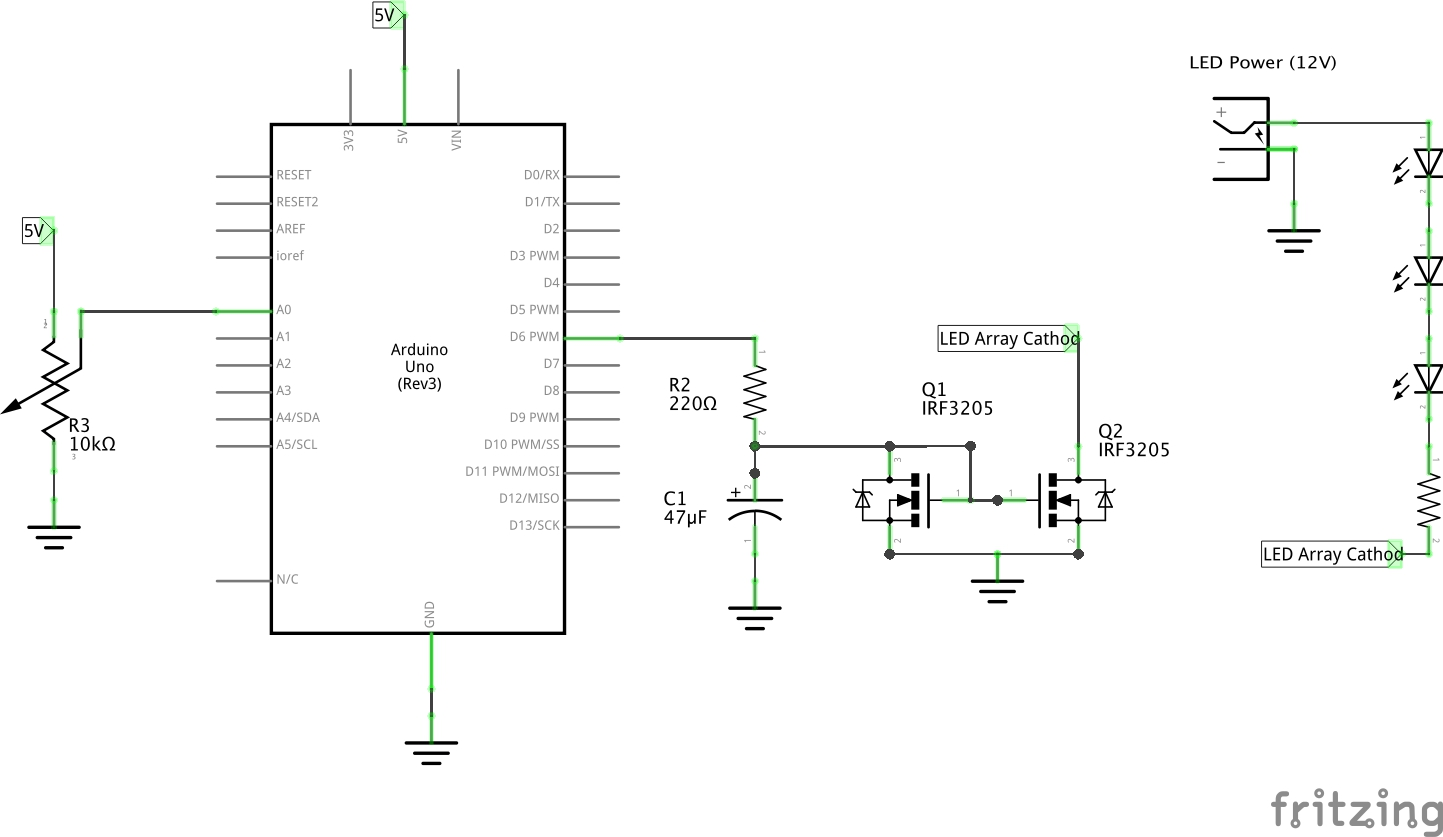Initial breadboard test with a single LED powered from the 5V rail: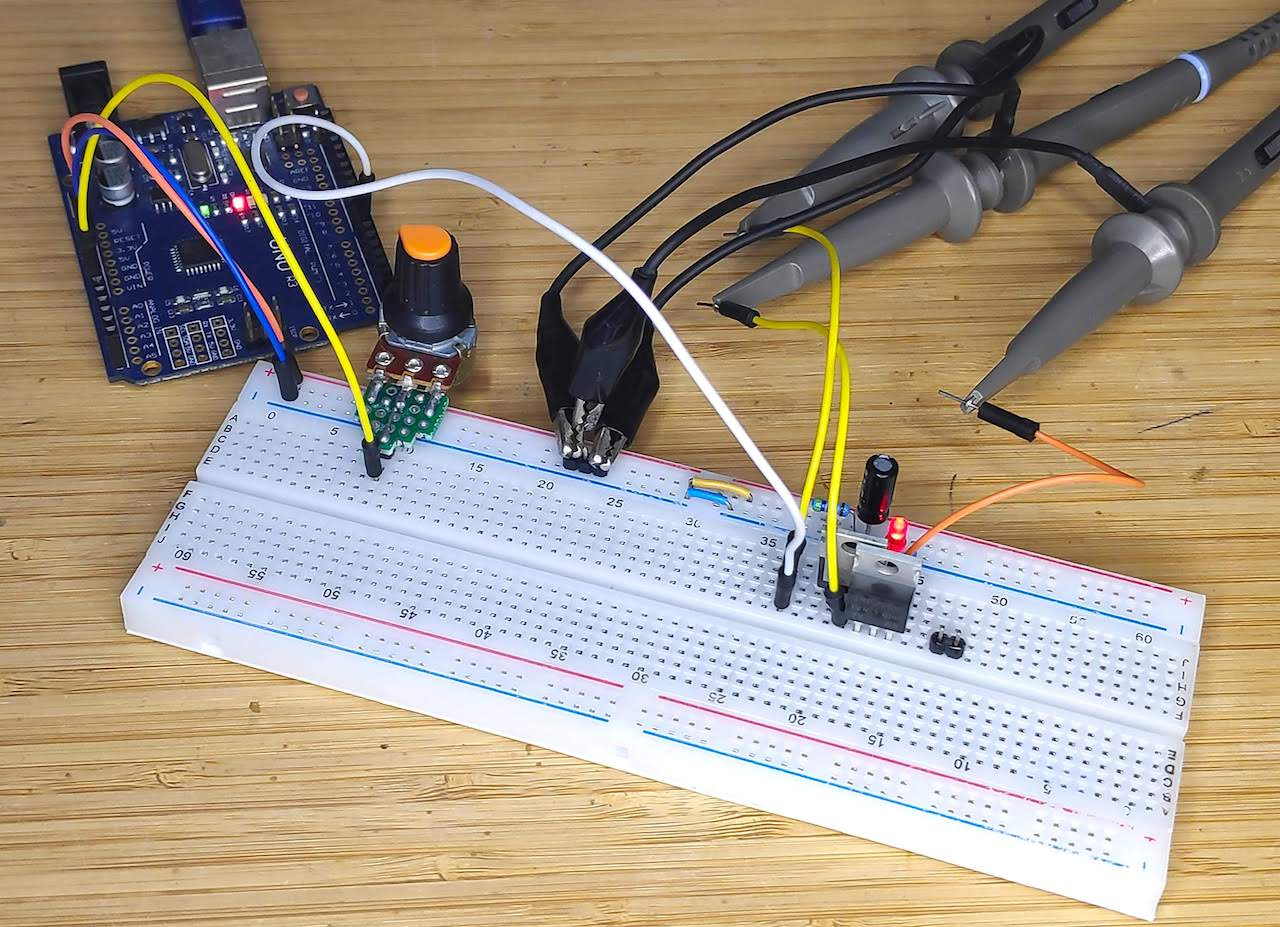## Performance

• CH1 (Yellow) - Pin 6 PWM output
• CH2 (Blue) - smoothed PWM signal at drain of Q1
• CH3 (Red) - voltage at drain of Q2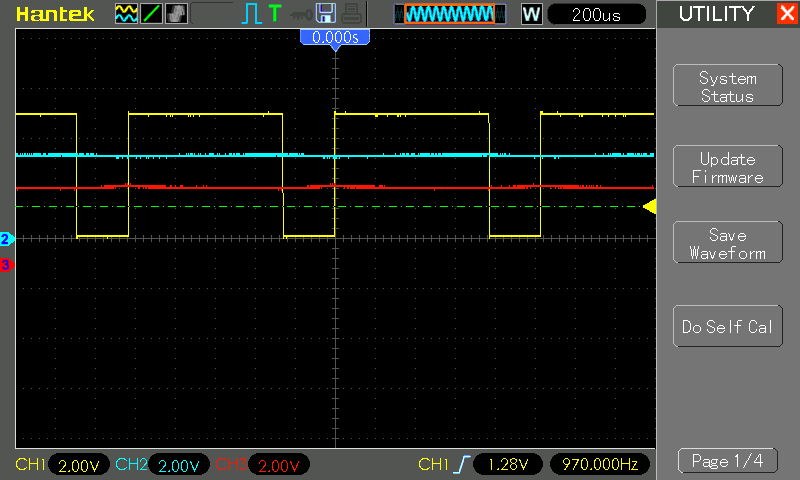## Code

See LedCurrentControl.ino for the sketch. Nothing sophisticated, simply a loop that:

The only tricky/non-obvious part is the scaling of the PWM output. The range is set between 120 and 255, as below 120 the LED is effectively off. Scaling to this range allows the pot to be effective over it’s full range.

## Conclusion and Limitations

This has demonstrated the effectiveness of PWM-controlled current mirror, however there are two obvious limitations of this approach in practice:

• a microcontroller can only safely provide a small current (typically 20mA) without additional circuitry to amplify the output. Beyond a few LEDs, the required LED current will likely be much larger.
• with large LED currents, a 1:1 current-mirror is very inefficient